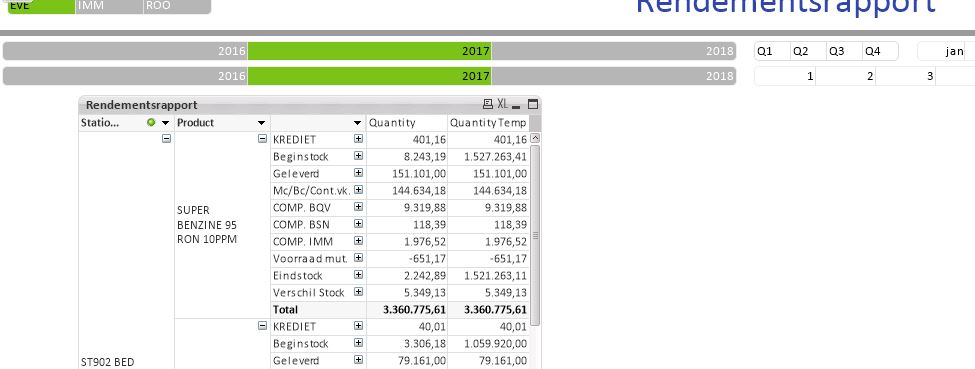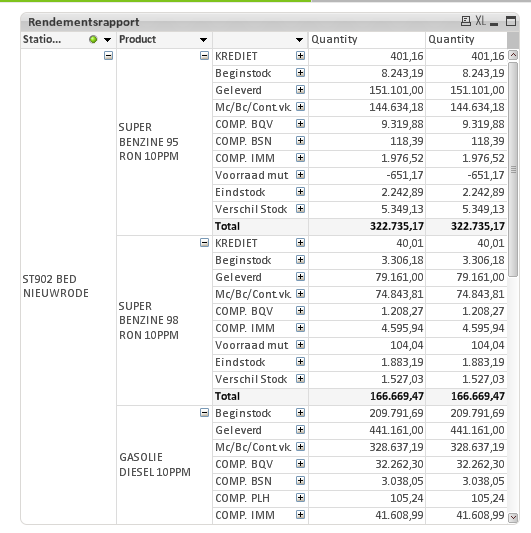# QlikView App Development

Discussion Board for collaboration related to QlikView App Development.

HighlightedPartner

## Total in pivot table not correct

Hi All,

I have a Pivot table with 3 dimensions and 1 measure

In the measure (quantity)I have this definition

if(W1TEXT = 'Beginstock',  sum({ \$ <KeyDatum = {\$(vMindate)}   >}W1HOEV), if(W1TEXT = 'Eindstock',  sum({ \$ <KeyDatum = {\$(vMaxdate)}   >}W1HOEV),sum(W1HOEV))).

The values in the table are correct.

Only the total is the value of sum(W1HOEV) (Quantity Temp)

Who can help me with the correct Total?

Regards,

KrisTags (2)
1 Solution

Accepted SolutionsMVP

## Re: Total in pivot table not correct

One option

RangeSum(

Sum({\$<KeyDatum = {\$(vMindate)}, W1TEXT *= {'Beginstock'}>}W1HOEV),

Sum({\$<KeyDatum = {\$(vMaxdate)}, W1TEXT *= {'Eindstock'}>}W1HOEV),

Sum({\$<W1TEXT -= {'Beginstock', 'Eindstock'}>}W1HOEV))

and other one is to

Sum(Aggr(if(W1TEXT = 'Beginstock',  sum({ \$ <KeyDatum = {\$(vMindate)}   >}W1HOEV), if(W1TEXT = 'Eindstock',  sum({ \$ <KeyDatum = {\$(vMaxdate)}   >}W1HOEV),sum(W1HOEV))), StationAlles, W1OMAR, W1TEXT, _CalYear, _CalMonth, _CalDay))

http://help.qlik.com/en-US/qlikview/November2017/Subsystems/Client/Content/ChartFunctions/NestedAggr...8 RepliesPartner

## Re: Total in pivot table not correct

Hi,

The pivot table is showing individual values per dimension. The most likely reason you are seeing these is that instances are duplicated (not unique) and is therefore summing up to a higher value.Partner

## Re: Total in pivot table not correct

Hi,

And how can I solve this issue?

The total mode is grayed out So I can't sit this to Sum of rows.

Can I do some thing in the expression?

Contributor

## Re: Total in pivot table not correctPartner

## Re: Total in pivot table not correct

Here is the app.

The total should be correct for every view.

If I only see 3 dimensions it should also be corect if possibleMVP

## Re: Total in pivot table not correct

One option

RangeSum(

Sum({\$<KeyDatum = {\$(vMindate)}, W1TEXT *= {'Beginstock'}>}W1HOEV),

Sum({\$<KeyDatum = {\$(vMaxdate)}, W1TEXT *= {'Eindstock'}>}W1HOEV),

Sum({\$<W1TEXT -= {'Beginstock', 'Eindstock'}>}W1HOEV))

and other one is to

Sum(Aggr(if(W1TEXT = 'Beginstock',  sum({ \$ <KeyDatum = {\$(vMindate)}   >}W1HOEV), if(W1TEXT = 'Eindstock',  sum({ \$ <KeyDatum = {\$(vMaxdate)}   >}W1HOEV),sum(W1HOEV))), StationAlles, W1OMAR, W1TEXT, _CalYear, _CalMonth, _CalDay))

http://help.qlik.com/en-US/qlikview/November2017/Subsystems/Client/Content/ChartFunctions/NestedAggr...Partner

## Re: Total in pivot table not correct

Thanks Sunny,

These both seem to work.

Which should I take?

regards,

KrisMVP

## Re: Total in pivot table not correct

I would suggest using the 1st option unless you see it causing problems...Partner

## Re: Total in pivot table not correct

Ok, Thanks for the quick help.